# An AM signal is represented by x(t)={20+4sin(500t)}cos(5000t). What will be the transmission efficiency? Express the answer in percentage.

Question
1 views

An AM signal is represented by x(t)={20+4sin(500t)}cos(5000t).

What will be the transmission efficiency? Express the answer in percentage.

check_circle

star
star
star
star
star
1 Rating
Step 1

The general expression of am amplitude modulated waveform can be expressed as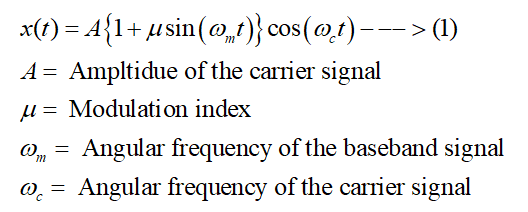The transmission efficiency of an amplitude modulated (AM) waveform is expressed as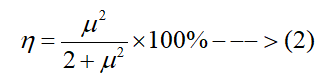Step 2

In this case, the expression of the amplitude modulated waveform is given as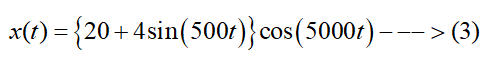The waveform expressed by equation (2) can be rewritten as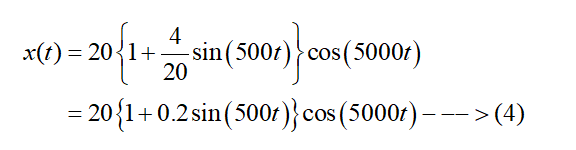Step 3

Comparing equation (1) and (3), it can be concluded that the value of the modulation index will be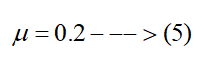...

### Want to see the full answer?

See Solution

#### Want to see this answer and more?

Solutions are written by subject experts who are available 24/7. Questions are typically answered within 1 hour.*

See Solution
*Response times may vary by subject and question.
Tagged in